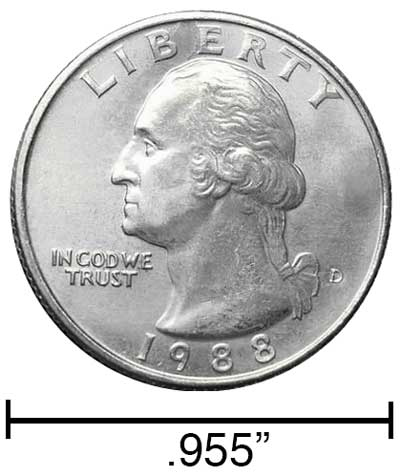# Inches to Micrometers Conversion

Enter the length in inches below to get the value converted to micrometers.

/
Results in Micrometers:1 in = 25,400 µm

## How to Convert Inches to MicrometersTo convert an inch measurement to a micrometer measurement, multiply the length by the conversion ratio.

Since one inch is equal to 25,400 micrometers, you can use this simple formula to convert:

micrometers = inches × 25,400

The length in micrometers is equal to the inches multiplied by 25,400.

For example, here's how to convert 5 inches to micrometers using the formula above.
5" = (5 × 25,400) = 127,000 µm

### How Many Micrometers are in an Inch?

There are 25,400 micrometers in an inch, which is why we use this value in the formula above.

1" = 25,400 µm

Our inch fraction calculator can add inches and micrometers together, and it also automatically converts the results to US customary, imperial, and SI metric values.

## Inches

An inch is a unit of linear length measure equal to 1/12 of a foot or 1/36 of a yard. Because the international yard is legally defined to be equal to exactly 0.9144 meters, one inch is equal to 2.54 centimeters.

The inch is a US customary and imperial unit of length. Inches can be abbreviated as in; for example, 1 inch can be written as 1 in.

Inches can also be denoted using the symbol, otherwise known as a double-prime. Often a double-quote (") is used instead of a double-prime for convenience. A double-prime is commonly used to express 1 in as 1″.The standard ruler has 12", and is a common measuring tool for measuring inches. They are also often measured using tape measures, which commonly come in lengths from 6' - 35'. Other types of measuring devices include scales, calipers, measuring wheels, micrometers, yardsticks, and even lasers.

## Micrometers

One micrometer is equal to one-millionth (1/1,000,000) of a meter, which is defined as the distance light travels in a vacuum in a 1/299,792,458 second time interval.

The micrometer, or micrometre, is a multiple of the meter, which is the SI base unit for length. In the metric system, "micro" is the prefix for 10-6. A micrometer is sometimes also referred to as a micron. Micrometers can be abbreviated as µm; for example, 1 micrometer can be written as 1 µm.

To get an idea of the actual physical length of a micrometer, one human hair is 40-50 µm thick, demonstrating how small this unit of measure is.

We recommend using a ruler or tape measure for measuring length, which can be found at a local retailer or home center. Rulers are available in imperial, metric, or combination with both values, so make sure you get the correct type for your needs.

Need a ruler? Try our free downloadable and printable rulers, which include both imperial and metric measurements.

## Inch to Micrometer Conversion Table

Inch measurements converted to micrometers
Inches Micrometers
0.0001" 2.54 µm
0.0002" 5.08 µm
0.0003" 7.62 µm
0.0004" 10.16 µm
0.0005" 12.7 µm
0.0006" 15.24 µm
0.0007" 17.78 µm
0.0008" 20.32 µm
0.0009" 22.86 µm
0.001" 25.4 µm
0.002" 50.8 µm
0.003" 76.2 µm
0.004" 101.6 µm
0.005" 127 µm
0.006" 152.4 µm
0.007" 177.8 µm
0.008" 203.2 µm
0.009" 228.6 µm
0.01" 254 µm
0.02" 508 µm
0.03" 762 µm
0.04" 1,016 µm
0.05" 1,270 µm
0.06" 1,524 µm
0.07" 1,778 µm
0.08" 2,032 µm
0.09" 2,286 µm
0.1" 2,540 µm
0.2" 5,080 µm
0.3" 7,620 µm
0.4" 10,160 µm
0.5" 12,700 µm
0.6" 15,240 µm
0.7" 17,780 µm
0.8" 20,320 µm
0.9" 22,860 µm
1" 25,400 µm

## References

1. National Institute of Standards and Technology, Checking the Net Contents of Packaged Goods, Handbook 133 - 2019 Edition, https://nvlpubs.nist.gov/nistpubs/hb/2019/NIST.HB.133-2019.pdf